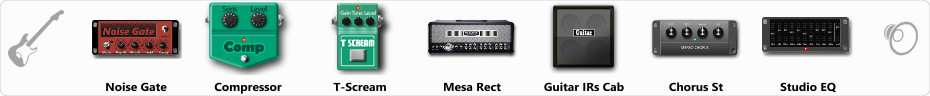# Mesa Boogie Dual Rectifier v3

Discussion in 'ToneLib-GFX presets' started by Serg10, Aug 28, 2019.

1. Mesa Boogie Dual Rectifier v3

Preset name: Mesa Boogie Dual Rectifier v3

Effects chain:Effect: "Noise Gate" (Dynamics / Filter), active - "yes"
"Detect" = Gtr In
"Mode" = Manual
"Depth" = 100
"Threshold" = 73
"Attack" = 0
"Hold" = 5
"Decay" = 5

Effect: "Compressor" (Dynamics / Filter), active - "yes"
"Sense" = 80
"Level" = 72

Effect: "T-Scream" (Overdrive / Distortion), active - "yes"
"Drive" = 10
"Tone" = 50
"Level" = 95

Effect: "Mesa Rect" (Amp simulators), active - "yes"
"Gain" = 50
"Bass" = 58
"Middle" = 74
"Treble" = 40
"Presence" = 93
"Master" = 60
"Output" = 50
"Level (dB)" = 0

Effect: "Guitar IRs Cab" (Cabinets), active - "yes"
"Model" = Peavey 5150 (4x12")
"Mic Position" = Middle
"Mic Distance" = Near
"Low Cut (Hz)" = 82
"Hi Cut (kHz)" = 12.0
"Mix" = 100
"Level (dB)" = 0

Effect: "Chorus St" (Modulation / Sfx), active - "yes"
"Speed" = 0.1
"Depth" = 80
"Center" = 5.5
"Mix" = 100

Effect: "Studio EQ" (Dynamics / Filter), active - "yes"
"31 Hz" = 0
"62 Hz" = 0
"125 Hz" = 0
"250 Hz" = 0
"500 Hz" = 0
"1 kHz" = -4
"2 kHz" = 10
"4 kHz" = 0
"8 kHz" = 0
"16 kHz" = 0
"above 16 kHz" = 0
"Level (dB)" = -4

Note: You will need to download and install the ToneLib-GFX software to use the preset.

File size:
665 bytes
Views:
4,675